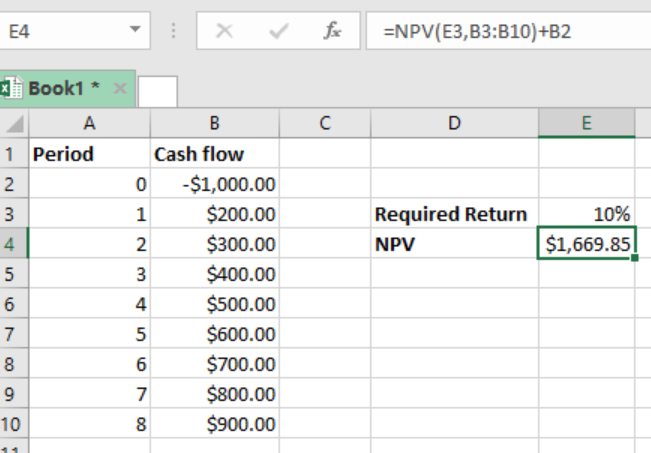Get instant live expert help with Excel or Google Sheets“My Excelchat expert helped me in less than 20 minutes, saving me what would have been 5 hours of work!”

#### Post your problem and you’ll get Expert help in seconds.

Your message must be at least 40 characters
Our professional Expert are available now. Your privacy is guaranteed.

# Excel NPV Function

If we want to calculate the net present value of an investment using a given discount rate, we can make use of the excel NPV function. This article will guide you on how to utilize this function to find the net present value.Figure 1: Using NPV function to find net present value

## General syntax of the formula

`=NPV (rate, value1, [value2]…)`

Where;

• Rate- refers to the discount rate over a single period
• Value1- this is the first value that represents the cash flow, it is mandatory
• Value2- refers to the second value that represents the cash flow, it is optional

## Understanding the formula

When supplied with the discount rate and a series of cash flows, the NPV function is able to calculate the net present value of an investment. Here, the rate is assumed to be annual, and is applied per each period. You should note that NPV ideally refers to the net value, but in real sense it is present value of uneven cash flows. You can still get the same answer if you remove the initial invested amount from the argument values that have been used in the NPV function.

### For you to work with this formula;

• The values must be arranged in a chronological order
• Values should be equally spaced in time and occur at the end of each given period.

## Instant Connection to an Expert through our Excelchat Service

Most of the time, the problem you will need to solve will be more complex than a simple application of a formula or function. If you want to save hours of research and frustration, try our live Excelchat service! Our Excel Experts are available 24/7 to answer any Excel question you may have. We guarantee a connection within 30 seconds and a customized solution within 20 minutes.

### Did this post not answer your question? Get a solution from connecting with the expert.Another blog reader asked this question today on Excelchat:## Subscribe to Excelchat.coAnother blog reader asked this question today on Excelchat: